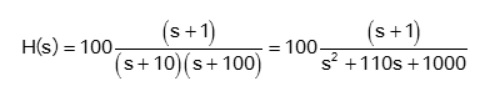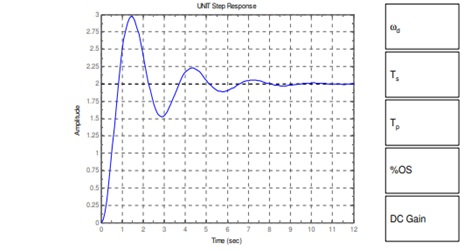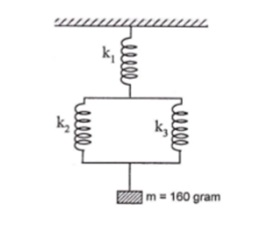We're Open

100% Pass and No Plagiarism Guaranteed

# Answer: Element 011: MOD005409 Assessment

Element 011: MOD005409 Assessment

Instructions to Students: Element 011: MOD005409 Assessment

2. The reflective parts for each question have 6 marks and limited to 50 words. This is about capturing concise/key conceptual understanding; exceeding word limit for this part will result in penalisation and your reflective writing will be marked out of 3
3. The data for some questions must be taken from your SID. Not complying to the instructions provided in each question, will result in mark reductions.
4. The deadline will be stated on the Canvas assignment page
5. This work will be submitted to Turnitin
6. Marks have been allocated individually to each question, adding to 100

Question 1:

Elaborate and Draw the Bode diagram for the following transfer function shown in Figure.Q1 through:

(a)  Showing transfer function for separate parts,

(2 marks)

(b)  Drawing the Bode diagram for each part,

(3 marks)

(c)  Drawing the overall Bode diagram.

(5 marks)Figure.Q1

Provide a reflective commentary underpinning the KEY conceptual elements in solving this problem (maximum 50 words, 6 Marks)

(Total 16 marks)

Question 2

(a) A system has a pair of complex conjugate poles p1 and p2 = -1+j2 and -1-j2 respectively and a single real zero z1 = -4 and a gain factor k = 3. Explain how to find the differential equation representing the system.

(5 marks)

(b) Explain and Identify the common terms in transient response characteristics of control system and specify the accepted limits in each transient response

(3 marks)

c) Explain by drawing the concepts of different types of damping types in relation to the natural angular velocity.

(3 marks)

Provide a reflective commentary underpinning the KEY conceptual elements in solving this problem (maximum 50 words, 6 Marks)

(Total Question Mark: 17)

Question 3

Discuss how you calculate the following step response and complete the table next to it according to the measurement results as shown on each axis:(9 marks)

Provide a reflective commentary underpinning the KEY conceptual elements in solving this problem (maximum 50 words, 6 Marks) (Total 16 marks)

Question 4

1. 1.    A mass m is held up by a force F0 sin(Ѡt) and connected to a spring and a damping coefficient c with the ground. The following parameters are known: the mass m = 100kg, the spring stiffness k = 700x103 N/m, the peak force F0 = 350 N. If the revolution speed is 300 RPM and the damping ratio is 0.2, find the followings:

(a) Displacement amplitude X                                                (6 marks)

(b) Transmissibility ratio TR = FT/F0                                                          (4 marks)

(c) Magnitude of transmitted force FT                                     (4 marks)

Provide a reflective commentary underpinning the KEY conceptual elements in solving this problem (maximum 50 words, 6 Marks)

(Total 20 marks)

Question 5

A top-loading washing machine executes the spin cycle at 4 rotations per second. The mass of the drum is 10 kg and it has a diameter of 0.6 m. The stiffness and damping coefficient of the mount are 379 N/m and 37.7 N.s/m respectively. If the mass of clothes is 5 kg. If the clothes are in the same place in one side of the drum, calculate:

(a) The damping frequency and the natural frequency                              (3 marks)

(b) the damping ratio                                                                                 (3 marks)

(c) Vibration amplitude                                                                              (3 marks)

(d) Transmitted force                                                                                 (3 marks)

Provide a reflective commentary underpinning the KEY conceptual elements in solving this problem (maximum 50 words, 6 Marks)

(Total 18 marks)

Question 6

(a) A 160-gram object attaches at one end of a spring and the change in length of the spring is 4 cm. What is the change in length of three springs connected in series and parallel, as shown in the figure below if the three springs have the same constant, k = 40 N/m. Take the gravity acceleration as 10 m/s2.(6 marks)

Provide a reflective commentary underpinning the KEY conceptual elements in solving this problem (maximum 50 words, 6 Marks)

(Total 13 marks)

End of Questions:

100% Plagiarism Free & Custom Written,The services provided by Assignment Experts UK are 100% original and custom written. We never use any paraphrasing tool, any software to generate content for e.g. Chat GPT and all other content writing tools. We ensure that the work produced by our writers is self-written and 100% plagiarism-free.

International House, 12 Constance Street, London, United Kingdom,
E16 2DQ

## STILL NOT CONVINCED?

We've produced some samples of what you can expect from our Academic Writing Service - these are created by our writers to show you the kind of high-quality work you'll receive. Take a look for yourself!